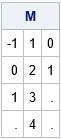In the SAS/IML language, you can only concatenate vectors that have conforming dimensions. For example, to horizontally concatenate two vectors X and Y, the symbols X and Y must have the same number of rows. If not, the statement Z = X || Y will produce an error: `ERROR: Matrices do not conform to the operation.`

The other day I wanted to concatenate multiple vectors of different sizes into a single matrix. I decided to use missing values to pad the "short" vectors. To make the operation reusable, I developed an IML module that accepts up to 16 different optional arguments. The module uses a few advanced techniques. This article presents the module and discusses programming techniques that you can use to systematically process all arguments passed to a SAS/IML module. Even if you never need to use the module, the techniques that implement the module are useful.

I wrote the module because I wanted to store multiple statistics of different sizes in a single matrix. For example, you can use this technique to store the data, parameter estimates, and the result of a hypothesis test. You can use a list to store the values, but for my application a matrix was more convenient. I've used a similar construction to store an array of matrices in a larger matrix.

### A module to concatenate vectors of different lengths

Although "parameter" and "argument" are often used interchangeably, for this article I will try to be consistent by using the following definitions. A parameter is a local variable in the declaration of the function. An argument is the actual value that is passed to function. Parameters are known at compile time and never change; arguments are known at run time and are potentially different every time the function is called.

Let's first see what the module does, then we'll discuss how it works. The module is defined as follows:

```proc iml; /* Pack k vectors into a matrix, 1 <= k <= 16. Each vector becomes a column. The vectors must be all numeric or all character. */ start MergeVectors(X1, X2=, X3=, X4=, X5=, X6=, X7=, X8=, X9=, X10=, X11=, X12=, X13=, X14=, X15=, X16= ); ParmList = "X1":"X16"; /* Names of params. Process in order. */ done = 0; /* flag. Set to 1 when empty parameter found */ type = type(X1); /* type of first arg; all args must have this type */ maxLen = 0; /* for character args, find longest LENGTH */ N = 0; /* find max number of elements in the args */   /* 1. Count args and check for consistent type (char/num). Stop at first empty arg */ do k = 1 to ncol(ParmList) until(done); arg = value( ParmList[k] ); /* get value from name */ done = IsEmpty(arg); /* if empty matrix, then exit loop */ if ^done then do; /* if not empty matrix... */ if type(arg)^= type then /* check type for consistency */ STOP "ERROR: Arguments must have the same type"; maxLen = max(maxLen,nleng(arg)); /* save max length of char matrices */ N = max(N,prod(dimension(arg))); /* save max number of elements */ end; end;   numArgs = k - 1; /* How many args were supplied? */ if type="N" then init = .; /* if args are numeric, use numeric missing */ else init = BlankStr(maxLen); /* if args are character, use character missing */ M = j(N, numArgs, init); /* allocate N x p matrix of missing values */   /* 2. Go through the args again. Fill i_th col with values of i_th arg */ do i = 1 to numArgs; arg = value( ParmList[i] ); /* get i_th arg */ d = prod(dimension(arg)); /* count number of elements */ M[1:d,i] = arg[1:d]; /* copy into the i_th column */ end; return( M ); /* return matrix with args packed into cols */ finish;   /* test the module */ M = MergeVectors(-1:1, T(1:4), 0:1); print M;```In the example, the function is passed three arguments of different sizes. The function returns a matrix that has three columns. The i_th column contains the values of the i_th parameter. The matrix contains four rows, which is the number of elements in the second argument. Missing values are used to pad the columns that have fewer than four elements.

### How to process all arguments to a function

The module in the previous section is defined to take one required parameter and 15 optional parameters. The arguments to the function are processed by using the following techniques:

• The ParmList vector contains the names of the parameters: ParmList = "X1":"X16".
• The module loops over the parameters and uses the VALUE function to get the value of the argument that is passed in.
• The ISEMPTY function counts the number of arguments that are passed in. At the same time, the module finds the maximum number of elements in the arguments.
• The module allocates a result matrix, M, that initially contains all missing values. The module iterates over the arguments and fills the columns of M with their values.

In summary, it is sometimes useful to pack several vectors of different sizes into a single matrix. But even if you never need this functionality, the structure of this module shows how to process an arbitrary number of optional arguments where each argument is processed in an identical manner.

The post Store vectors of different lengths in a matrix appeared first on The DO Loop.